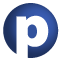# immutable

### Type Members

1. #### class ::[B](hd: B, tl: List[B]) extends List[B] with Product

A non empty list characterized by a head and a tail

2. #### class BitSet extends Set[Int] with BitSet with BitSetLike[BitSet]

A base class for immutable bit sets

3. #### class HashMap[A, +B] extends Map[A, B] with MapLike[A, B, HashMap[A, B]] with HashTable[A]

This class implements immutable maps using a hash table

4. #### class HashSet[A] extends Set[A] with GenericSetTemplate[A, HashSet[A][A]] with SetLike[A, HashSet[A]] with FlatHashTable[A]

This class implements immutable sets using a hash table

5. #### trait IndexedSeq[+A] extends Seq[A] with IndexedSeq[A] with GenericTraversableTemplate[A, IndexedSeq[A][A]] with IndexedSeqLike[A, IndexedSeq[A]]

A subtrait of `collection`

``` ```
6. ``` class IntMap[+T] extends Map[Int, T] with MapLike[Int, T, IntMap[T]] Specialised immutable map structure for integer keys, based on trait Iterable[+A] extends Traversable[A] with Iterable[A] with GenericTraversableTemplate[A, Iterable[A][A]] with IterableLike[A, Iterable[A]] A subtrait of scala ```
7. ``` trait LinearSeq[+A] extends Seq[A] with LinearSeq[A] with GenericTraversableTemplate[A, LinearSeq[A][A]] with LinearSeqLike[A, LinearSeq[A]] A subtrait of collection ```
8. ``` class List[+A] extends LinearSeq[A] with Product with GenericTraversableTemplate[A, List[A][A]] with LinearSeqLike[A, List[A]] A class for immutable linked lists representing ordered collections of elements of type ```
9. ``` class ListMap[A, +B] extends Map[A, B] with MapLike[A, B, ListMap[A, B]] This class implements immutable maps using a list-based data structure ```
10. ``` class ListSet[A] extends Set[A] with GenericSetTemplate[A, ListSet[A][A]] with SetLike[A, ListSet[A]] This class implements immutable sets using a list-based data structure ```
11. ``` class LongMap[+T] extends Map[Long, T] with MapLike[Long, T, LongMap[T]] Specialised immutable map structure for long keys, based on trait Map[A, +B] extends Iterable[(A, B)] with Map[A, B] with MapLike[A, B, Map[A, B]] ```
12. ``` trait MapLike[A, +B, +This <: MapLike[A, B, This] with Map[A, B]] extends MapLike[A, B, This] A generic template for immutable maps from keys of type A to values of type B ```
13. ``` trait MapProxy[A, +B] extends Map[A, B] with MapProxyLike[A, B, Map[A, B]] This is a simple wrapper class for class NumericRange[+T] extends IndexedSeq[T] NumericRange is a more generic version of the Range class which works with arbitrary types ```
14. ``` class PagedSeq[T] extends IndexedSeq[T] An implementation of lazily computed sequences, where elements are stored in pages, i ```
15. ``` class Queue[+A] extends Seq[A] Queue objects implement data structures that allow to insert and retrieve elements in a first-in-first-out (FIFO) manner ```
16. ``` class Range extends IndexedSeq[Int] The Range class represents integer values in range [start;end) with non-zero step value step ```
17. ``` class RedBlack[A] extends AnyRef ```
18. ``` trait Seq[+A] extends Iterable[A] with Seq[A] with GenericTraversableTemplate[A, Seq[A][A]] with SeqLike[A, Seq[A]] A subtrait of collection ```
19. ``` trait Set[A] extends Iterable[A] with Set[A] with GenericSetTemplate[A, Set[A][A]] with SetLike[A, Set[A]] A generic trait for immutable sets ```
20. ``` trait SetProxy[A] extends Set[A] with SetProxyLike[A, Set[A]] This is a simple wrapper class for trait SortedMap[A, +B] extends Map[A, B] with SortedMap[A, B] with MapLike[A, B, SortedMap[A, B]] with SortedMapLike[A, B, SortedMap[A, B]] A map whose keys are sorted ```
21. ``` trait SortedSet[A] extends Set[A] with SortedSet[A] with SortedSetLike[A, SortedSet[A]] A sorted set ```
22. ``` class Stack[+A] extends LinearSeq[A] with GenericTraversableTemplate[A, Stack[A][A]] with LinearSeqLike[A, Stack[A]] This class implements immutable stacks using a list-based data structure ```
23. ``` class Stream[+A] extends LinearSeq[A] with GenericTraversableTemplate[A, Stream[A][A]] with LinearSeqLike[A, Stream[A]] The class Stream implements lazy lists where elements are only evaluated when they are needed ```
24. ``` trait StringLike[+Repr] extends IndexedSeqLike[Char, Repr] with Ordered[String] ```
25. ``` class StringOps extends StringLike[String] ```
26. ``` trait Traversable[+A] extends Traversable[A] with GenericTraversableTemplate[A, Traversable[A][A]] with TraversableLike[A, Traversable[A]] with Immutable A subtrait of collection ```
27. ``` class TreeHashMap extends AnyRef ```
28. ``` class TreeMap[A, +B] extends RedBlack[A] with SortedMap[A, B] with SortedMapLike[A, B, TreeMap[A, B]] with MapLike[A, B, TreeMap[A, B]] This class implements immutable maps using a tree ```
29. ``` class TreeSet[A] extends RedBlack[A] with SortedSet[A] with SortedSetLike[A, TreeSet[A]] This class implements immutable sets using a tree ```
30. ``` class Vector[+A] extends Seq[A] with GenericTraversableTemplate[A, Vector[A][A]] with SeqLike[A, Vector[A]] with VectorPointer[A] ```
31. ``` class VectorBuilder[A] extends Builder[A, Vector[A]] with VectorPointer[A] ```
32. ``` class VectorIterator[+A] extends Iterator[A] with VectorPointer[A] ```
33. ``` class WrappedString extends IndexedSeq[Char] with StringLike[WrappedString] with Proxy ```
``` ```
``` Value Members object BitSet extends BitSetFactory[BitSet] A factory object for bitsets object HashMap extends ImmutableMapFactory[HashMap[A, B][A, B]] A factory object for immutable HashMaps object HashSet extends SetFactory[HashSet[A][A]] A factory object for immutable HashSets object IndexedSeq extends SeqFactory[IndexedSeq[A][A]] object IntMap extends AnyRef object Iterable extends TraversableFactory[Iterable[A][A]] A factory object for the trait Iterable object LinearSeq extends SeqFactory[LinearSeq[A][A]] object List extends SeqFactory[List[A][A]] \$factoryInfo object ListMap extends ImmutableMapFactory[ListMap[A, B][A, B]] The canonical factory of object ListSet extends SetFactory[ListSet[A][A]] The canonical factory of object LongMap extends AnyRef object Map extends ImmutableMapFactory[Map[A, B][A, B]] object Nil extends List[Nothing] with Product The empty list object NumericRange extends AnyRef object PagedSeq extends AnyRef The PagedSeq object defines a lazy implementations of a random access sequence object Queue extends AnyRef object Range extends AnyRef object Seq extends SeqFactory[Seq[A][A]] object Set extends SetFactory[Set[A][A]] object SortedMap extends ImmutableSortedMapFactory[SortedMap[A, B][A, B]] object SortedSet extends ImmutableSortedSetFactory[SortedSet[A][A]] object Stack extends SeqFactory[Stack[A][A]] object Stream extends SeqFactory[Stream[A][A]] The object Stream provides helper functions to manipulate streams object StringLike extends AnyRef object Traversable extends TraversableFactory[Traversable[A][A]] A factory object for the trait Traversable object TreeMap extends ImmutableSortedMapFactory[TreeMap[A, B][A, B]] The canonical factory of object TreeSet extends SortedSetFactory[TreeSet[A][A]] The canonical factory of object Vector extends SeqFactory[Vector[A][A]] object WrappedString extends AnyRef ```
``` ```